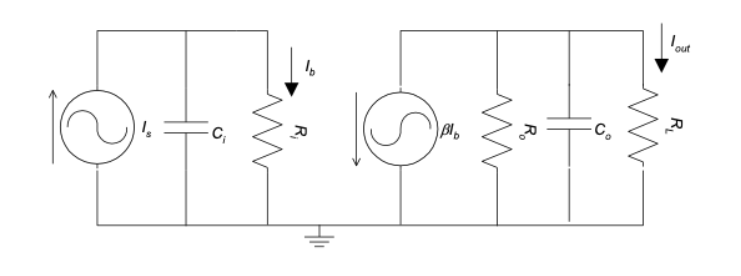Home / Expert Answers / Electrical Engineering / ri-2mw-ro-10mw-rl-1mw-ci-50nf-co-40pf-b-100a-derive-the-transfer-function-for-your-circui-pa160

# (Solved): Ri = 2MW, Ro= 10MW, RL= 1MW. Ci =50nF, Co=40pF, b=100A: Derive the Transfer Function for your circui ...Ri = 2MW, Ro= 10MW, RL= 1MW. Ci =50nF, Co=40pF, b=100

A: Derive the Transfer Function for your circuit in pronumerals H(s)=Iout(s)/Is(s) for the circuit

B: Substitute the numerical values and express the Transfer Function numerically:

We have an Answer from Expert

Given Information:

Ri = 2MW,
Ro= 10MW,
RL= 1MW,
Ci =50nF,
Co=40pF,
b=100

The circuit is shown below:

Given circuit with all information.

We have an Answer from Expert